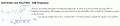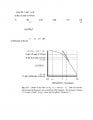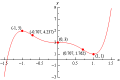# 2nd-Order Filter Corner Frequency second order cut-off frequency

#### ntetlow

Joined Jul 12, 2019
52
2nd-Order Low Pass Filter -3dB FrequencyAbove is a formula extracted from electronicstutorials . It refers to a second order low pass filter to calculate the actual cut off frequency for any level (n) of low pass fillers. The normal cut of frequency given by the formulaCan anyone explain this to me , I assume the '1' in the formula is outside the square root symbol?Mod: Opened the image

Last edited by a moderator:

#### bertus

Joined Apr 5, 2008
21,944
Hallo,

I do not see the posted images.
Upload the images to the forum.

Bertus

#### ntetlow

Joined Jul 12, 2019
52
Hallo,

I do not see the posted images.
Upload the images to the forum.

Bertus
If you click 'EDIT' you will see them.

#### Papabravo

Joined Feb 24, 2006
18,812
2nd-Order Low Pass Filter -3dB FrequencyAbove is a formula extracted from electronicstutorials . It refers to a second order low pass filter to calculate the actual cut off frequency for any level (n) of low pass fillers. The normal cut of frequency given by the formulaCan anyone explain this to me , I assume the '1' in the formula is outside the square root symbol?View attachment 220170

Mod: Opened the image
The explanation is that, although close to each other, the corner frequency and the the 3 dB down frequency are not exactly the same. The corner frequency is actually a drawing aid because it provides an asymptote for the filter rolloff.

#### ntetlow

Joined Jul 12, 2019
52
The explanation is that, although close to each other, the corner frequency and the the 3 dB down frequency are not exactly the same. The corner frequency is actually a drawing aid because it provides an asymptote for the filter rolloff.
Sorry but it is the formula that I am concerned with. I want to know how it is derived. An example would also be nice.

#### crutschow

Joined Mar 14, 2008
30,119
If you click 'EDIT' you will see them.
No, they are X'd out.

I believe the 1 is beneath the square-root symbol.

#### Papabravo

Joined Feb 24, 2006
18,812
Sorry but it is the formula that I am concerned with. I want to know how it is derived. An example would also be nice.
The formula is derived from the transfer function of the filter. What kind of filter is it? Butterworth, Bessel, chebyshev, Something else?
Alternatively where are the poles of this 2nd order filter? From the poles we can derive the transfer function.

#### ntetlow

Joined Jul 12, 2019
52
The formula is derived from the transfer function of the filter. What kind of filter is it? Butterworth, Bessel, chebyshev, Something else?
Alternatively where are the poles of this 2nd order filter? From the poles we can derive the transfer function.
If you look in electronics-tutorials.ws website you'll find the piece under low pass filters , second level. It is just an ordinary 2 cap 2 resistor circuit.

#### Papabravo

Joined Feb 24, 2006
18,812
If you look in electronics-tutorials.ws website you'll find the piece under low pass filters , second level. It is just an ordinary 2 cap 2 resistor circuit.
So what is the transfer function?

#### ntetlow

Joined Jul 12, 2019
52

#### Papabravo

Joined Feb 24, 2006
18,812

#### Papabravo

Joined Feb 24, 2006
18,812
Because of the following:

In practice, cascading passive filters together to produce larger-order filters is difficult to implement accurately as the dynamic impedance of each filter order affects its neighbouring network. However, to reduce the loading effect we can make the impedance of each following stage 10x the previous stage, so R2 = 10 x R1 and C2 = 1/10th C1.

The derivation of the transfer function is less straightforward in this case because the second stage loads the first. The strategy above may be used to mitigate that effect. The derivation of the actual transfer function comes from writing the node equations, and observing how the sections interact with each other. This leads to an expression for the magnitude of the output with respect to the magnitude of the input as functions of frequency which can then be solved for particular values, like 1/√2 = .707.

•Delta Prime

#### Papabravo

Joined Feb 24, 2006
18,812
Thank you for the precise link

#### ntetlow

Joined Jul 12, 2019
52
Because of the following:

In practice, cascading passive filters together to produce larger-order filters is difficult to implement accurately as the dynamic impedance of each filter order affects its neighbouring network. However, to reduce the loading effect we can make the impedance of each following stage 10x the previous stage, so R2 = 10 x R1 and C2 = 1/10th C1.

The derivation of the transfer function is less straightforward in this case because the second stage loads the first. The strategy above may be used to mitigate that effect. The derivation of the actual transfer function comes from writing the node equations, and observing how the sections interact with each other. This leads to an expression for the magnitude of the output with respect to the magnitude of the input as functions of frequency which can then be solved for particular values, like 1/√2 = .707.
Thank you for this. However the formula must have come from somewhere. I have searched the web and cannot find it anywhere. When things are so poorly explained as in this case my curiosity is roused and I cannot make sense of the maths at all.

#### Papabravo

Joined Feb 24, 2006
18,812
Thank you for this. However the formula must have come from somewhere. I have searched the web and cannot find it anywhere. When things are so poorly explained as in this case my curiosity is roused and I cannot make sense of the maths at all.
What you have to do for yourself is write the node equation to determine the transfer function. Then derive the result you are looking for. Can it be so hard for you to do that?

#### Delta Prime

Joined Nov 15, 2019
1,311
However the formula must have come from somewhere
hello thereCorner frequency, -3db , 0.707.
What you see below is a visual representation of what has already been discussed sorry for the repetition. Can you see how they relate to each other graphically.It doesn't matter what type of filter High pass low pass band pass pick one the principle is the same.

Last edited:

#### Delta Prime

Joined Nov 15, 2019
1,311
In practice, cascading passive filters together to produce larger-order filters is difficult to implement accurately as the dynamic impedance of each filter order affects its neighbouring network. However, to reduce the loading effect we can make the impedance of each following stage 10x the previous stage, so R2 = 10 x R1 and C2 = 1/10th C1
Commit this quote to memory! Then you will be on your way to design what you wish.